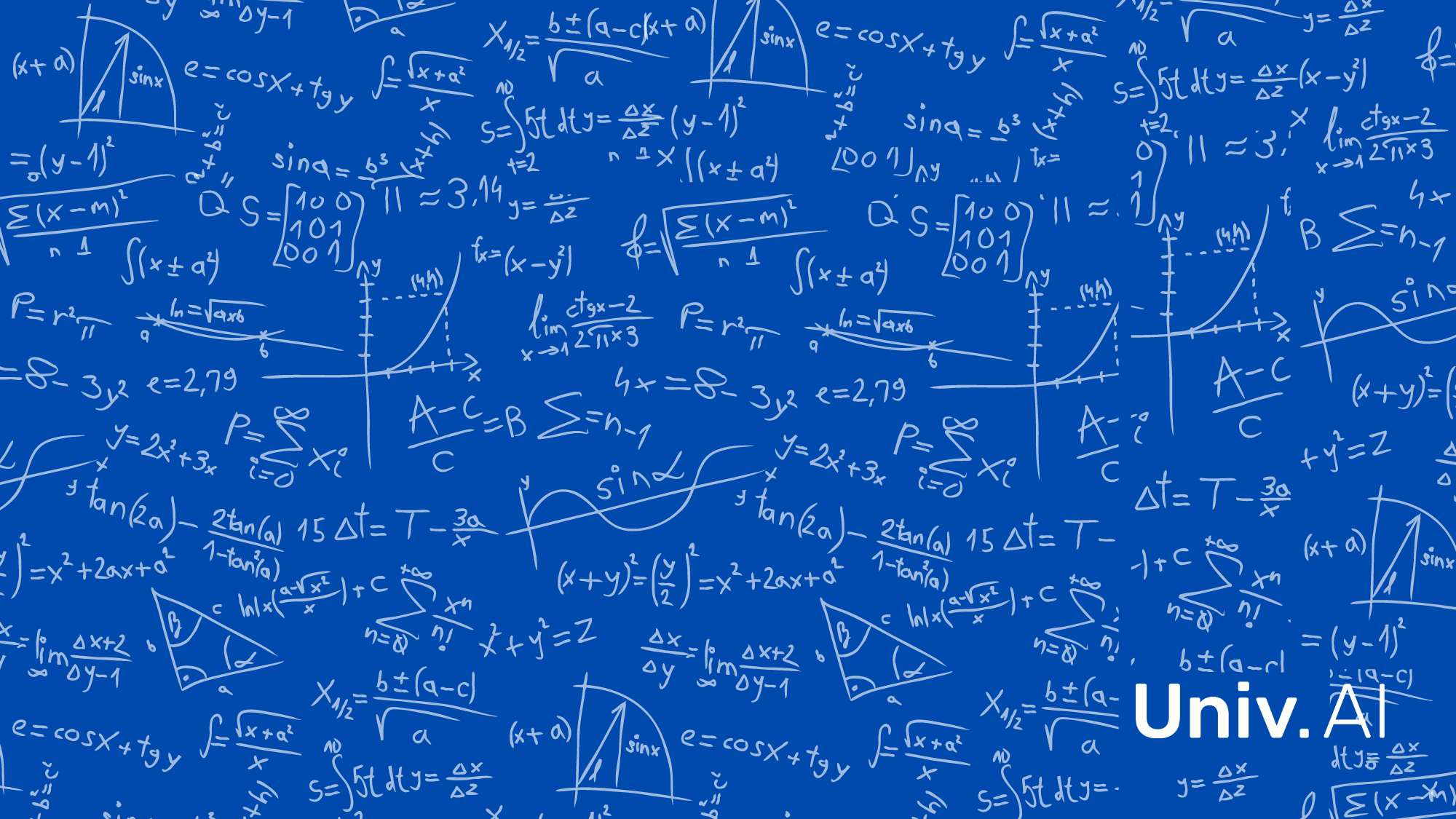## Machine Learning Prerequisites: Calculus

##### Most of us are familiar with calculus as all the stuff which involves differentiation and integration. But what does it mean in the realm of machine learning? Let's find out more.In Latin, ‘calculus’ means a small pebble. The word became associated with computation because the Romans did arithmetic with piles of stones. Today, calculus has evolved to become a branch which deals with much more than basic arithmetic.

Simply put, it describes a branch of mathematics that looks at functions as being made up of infinitesimally small pieces. Differential calculus cuts mathematical functions up into small pieces, whereas integral calculus joins these small pieces together. By analyzing how these small pieces are arranged, we can understand how functions vary. In this way, calculus is described as the study of continuous change.

Calculus plays an integral (pun intended) role in machine learning. Calculus for machine learning is often overlooked because deep learning libraries such as PyTorch conceal the underlying calculus formulae that make things work. If you want to make sense of what goes on under the hood or understand research papers discussing the latest advances in machine learning, you’ll need to have a solid grasp of the basics of calculus.

But how is calculus used in machine learning? If you're already thinking about gradient descent, you’re ‘rolling’ in the right direction!

Consider a linear regression problem of fitting a straight line to a dataset. The straight line could be represented as:
$y=mx+b$
where $y$ is the predictor, $m$ is the slope, $x$ is the input and $b$ is the y-intercept.

How do we find which of the above lines fit our data the best?

A standard approach to solving this type of problem is to define a cost or error function( E) which can be used as an indicator for the goodness of fit. The error, in this case, would be:

$E = \frac{1}{n} \sum_{i=1}^{n} (y_{i} - (mx_{i}+b))^2$

Now, intuitively, the right set of values for m and c are those which minimize this error function.

One prescribed method for minimizing this error function is gradient descent.
Gradient descent can be visualised as a ball rolling down a hill. The “hill” in this case is the 3D curve obtained by plotting the error function for every value of m and c.  The gradient at any point on this curve points to the direction of maximum change. This means that moving against the gradient pushes us closer to the minimum of the error function.

To compute the gradient, we will need to differentiate the error function. Since the function is defined by two variables (m and b), this is a multivariate calculus problem that requires computing the partial derivatives with respect to the two variables
These derivatives work out to be:

$m’ = \frac{\partial E}{\partial m} = \frac{-2}{n} \sum_{i=1}^{n} x_{i}(y_{i} - (mx_{i}+b))$

$b’ = \frac{\partial E}{\partial b} = \frac{-2}{n} \sum_{i=1}^{n} (y_{i} - (mx_{i}+b))$

Now, we update the current value of m and c using the following equation:

$m = m -(\alpha*m’)$

$b = b - (\alpha*b’)$

where $\alpha$ is the learning rate that describes the rate with which the values change.

This process is repeated until our error function is a small value. The value of m and c that we are left with now will be the optimum values for the best fitting line.

Calculus is not only limited to gradient computation. I’m sure you all have heard about backpropagation or generative adversarial networks. All of these have their foundations in calculus!

In machine learning, more often than not we deal with functions that contain hundreds of variables and degrees of freedom. In situations like this, it is helpful to utilize linear algebra, bringing in matrix calculus concepts such as the jacobian and hessian matrix where variables and gradients are represented with vectors and matrices.

###### Where to begin?

We have collated the right set of resources that make calculus easy for you:

Khan Academy's multivariable calculus lecture series is taught by Grant Sanderson from 3Blue1Brown. If you are a beginner looking for an introductory course in calculus, this course is for you! It answers “but why?” questions and gives you a good start to dive deeper into these concepts.

Essence of Calculus - 3Blue1Brown
The Essence of Calculus playlist contains 12 video lectures by Grant Sanderson. The lectures teach some important basics of calculus that are required in Data Science.

Essence of Calculus gets to the heart of this core mathematical study in one binge-watchable series of lessons. Grant’s distinctive animation-and-visuals style and friendly approach makes learning calculus not only intuitive but also fun!

Multivariable Calculus - MIT OpenCourseWare
This course is designed for undergraduate students and teaches calculus for beginners. It covers differential, integral and vector calculus for multivariable functions. The course is a second part to Single Variable Calculus which can be done if you are not familiar with the basics of one-variable calculus. Topics include vectors and matrices, parametric curves, partial derivatives, double and triple integrals, and vector calculus in 2- and 3-space. The material has been organized to promote self-study.

Multivariable Mathematics - Theodore Shifrin
Multivariable Mathematics combines linear algebra and multivariable calculus in a rigorous approach. The material is integrated to emphasize the role of linearity in all of calculus and the recurring theme of implicit versus explicit that persists in linear algebra and analysis. With a prior decent knowledge in these topics, this book provides an excellent view of matrix calculus. If you're up for a good challenge I would highly recommend this book because the exercises will definitely make you think.